# What are bending stiffness units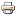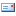In technical mechanics *, rigidity is a parameter that describes the resistance a body can provide against deformation due to external influences (torque or force). As a result, the rigidity depends on two factors: the geometry of the body and its material. A distinction is generally made between different stiffnesses such as elongation, torsion and bending stiffness. The distinction depends on the type of load. The reciprocal of stiffness is compliance.

### Types of stiffness in mechanics

Rigidity consists of two values: a geometry and a material term. The question of which size is used for the material (elasticity or shear modulus) depends on the load. This, in turn, is generated by the load. The stiffnesses are noted in such a way that the deformation variables can be identified - e.g. elongations and no changes in length. The background is simple: the stiffness belongs to the cross-section of the object. The cross-sectional geometry can change over the total length (such as with a bottle), so that a multiplication by the length would lead to errors.

### The tensile stiffness

The product of the modulus of elasticity E (material in the direction of load) and the cross-sectional area A (perpendicular to the direction of load) gives rise to the tensile stiffness in mechanics. Your formula is therefore:However, this formula refers to a free transverse contraction of the cross-section. However, if the transverse contraction is limited, the transverse contraction-impaired module must be used. For this, the modulus of elasticity is left out.

The elongation of the body is indicated by the letter ε. It is inversely proportional to the tensile stiffness and normal proportional to the normal force acting on it, which is described by the letter F. The following formula follows: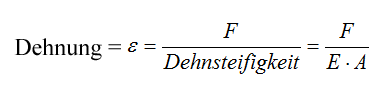### The bending stiffness

The product of the area moment of inertia I and the modulus of elasticity E is the so-called flexural rigidity. It should be noted that the geometrical moment of inertia is essentially dependent on the cross-sectional shape. It follows: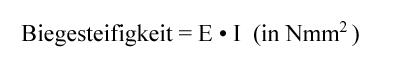In addition to the flexural rigidity, the storage conditions and the length of a component that is being bent are also decisive for the extent to which it can be deflected. The deflection is called the curvature κ. κ is proportional to the applied bending moment MB.. The curvature is also inversely proportional to the bending stiffness.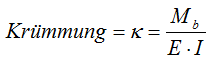### The torsional stiffness

The torsional stiffness is sometimes also described as torsional stiffness. It is the result of the product of the associated torsional moment of inertia I.T as well as the material thrust module G. IT is to be related to the axis around which the component is twisted.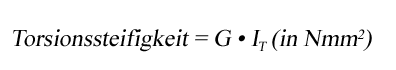It should be noted that IT does not generally correspond to the polar cross-sectional area moment of inertia Ip. This is only the case with circular cross-sections and with circular ring cross-sections. For I.T A closed formula can only be used in special cases.

For the question of how much a component twists under a load, next to I.T also on the length L and on the storage conditions. The twist per L-unit is called twist or twist (ʋ '). The twist is proportional to the torsional moment M.T and inversely proportional to the torsional stiffness. It follows:### Rigidity and strength

It should also be noted that stiffness must not be confused with strength *. Strength is a measure of how great the load a material can bear. It is therefore a limit value that can easily be researched in data sheets. An example of strength is tensile strength.

The rigidity, however, depends on the elasticity of the material and the shape and size of the cross-sectional area. The stiffness is consequently in no way dependent on the strength.

=> Stiffness ≠ strength